﻿ Optimal Controller for Wind Energy Conversion Systems

### Optimal Controller for Wind Energy Conversion SystemsOPEN ACCESSPEER-REVIEWED

## Optimal Controller for Wind Energy Conversion Systems

Hossein Nasir Aghdam1, Farzad Allahbakhsh1,1Department of Engineering, Ahar Branch, Islamic Azad University, Ahar, Iran

### Abstract

Increasing of word demand load is caused a new Distributed Generation (DG) enter to power system. One of the most renewable energy is the Wind Energy Conversion System (WECS), which is connected to power system using power electronics interface or directly condition. In this paper an optimal Lead-Lag controller for wind energy conversion systems (WECS) has been developed. The optimization technique is applied to Lead-Lag optimal controller in order to control of the most important types of wind system with doubly Fed Induction Generator is presents. Nonlinear characteristics of wind variations as plant input, wind turbine structure and generator operational behavior demand for high quality optimal controller to ensure both stability and safe performance. Thus, Honey Bee Mating Optimization (HBMO) is used for optimal tuning of Lead-Lag coefficients in order to enhance closed loop system performance. In order to use this algorithm, at first, problem is written as an optimization problem which includes the objective function and constraints, and then to achieve the most desirable controller, HBMO algorithm is applied to solve the problem. In this study, the proposed controller first is applied to two pure mathematical plants, and then the closed loop WECS behavior is discussed in the presence of a major disturbance. Simulation results are done for various loads in time domain, and the results show the efficiency of the proposed controller in contrast to the previous controllers.

### At a glance: Figures

1234
Prev Next

• Aghdam, Hossein Nasir, and Farzad Allahbakhsh. "Optimal Controller for Wind Energy Conversion Systems." Sustainable Energy 2.2 (2014): 57-62.
• Aghdam, H. N. , & Allahbakhsh, F. (2014). Optimal Controller for Wind Energy Conversion Systems. Sustainable Energy, 2(2), 57-62.
• Aghdam, Hossein Nasir, and Farzad Allahbakhsh. "Optimal Controller for Wind Energy Conversion Systems." Sustainable Energy 2, no. 2 (2014): 57-62.

 Import into BibTeX Import into EndNote Import into RefMan Import into RefWorks

### 1. Introduction

According to increasing consumption of fossil fuels in recent years and their limited resources, it is very vital to use unlimited natural energy resources such as water, wind and sun. A very common application of such resources is electrical energy generation. Water and wind energy are mostly used to rotate shafts of prime movers to generate energy [1, 2].

WECS are very challenging from the control system. In fact, wind turbine control enables a better use of the turbine capacity as well as the alleviation of aerodynamic and mechanical loads, which reduce the useful life of the installation .

Various approaches are introduced in literature in order to control WECS. In  a combined adaptive supervisory control approach is introduced which has a radial basis Zfunction adaptive controller and a simple supervisor. In  a PID controller is proposed in which PID parameters are tuned via a wavelet neural network that is a single layer network with wavelets of type RASP1 as hidden nodes. This network proposes a pre specified model structure to identify the unknown plant. In [6, 7] a self tuning control method for wind energy conversion system is introduced using wavelet networks.

Many predictive control algorithms were proposed during the last decades, such as dynamic matrix control (DMC), model algorithmic control (MAC) and generalized predictive control (GPC). Although they can obtain high performance of the controlled system, few of them have been realized in electrical machine or drive application due to the heavy calculation power required by them . Improving the reactive power compensation and active filtering capability of a Wind Energy Conversion System is proposed in . The proposed algorithm is applied to a Doubly Fed Induction Generator (DFIG) with a stator directly connected to the grid and a rotor connected to the grid through a back-to-back AC-DC-AC PWM converter. It has been shown also that the proposed strategy allows an operating full capacity of the RSC in terms of reactive power compensation and active filtering. Ref  describes a novel teaching experience using wind energy as the starting point for understanding power electronics and electrical machines. The results point out the wide variety of concepts involved in the course, the numerous competences that it can enhance, and its positive reception by learners. An analysis tool is proposed to estimate the theoretical control limit of the RSC in suppressing the short circuit rotor currents during grid faults in . To execute the analysis, a simplified DFIG model with decoupled stator and rotor fluxes is presented; the low-voltage ride through (LVRT) problem can be formulated as an optimization problem, which intends to suppress the rotor winding currents with voltage constraints. The results are expected to help the manufacturers to assess and improve their RSC controllers or LVRT measures.

In this paper an optimal-tuning Lead-Lag controller for a WECS is proposed based on Honey Bee Mating Optimization (HBMO) algorithm. So far none of the mentioned approaches in the literature analyzed the WECS in the presence of external disturbances. But, in this study, a major disturbance is applied to the close loop WECS and then system performance is analyzed. Furthermore, it will be shown that the system response in the proposed controller is considerably faster while no fluctuations are mounted on the control or output signals.

This paper is organized as follows: in section 2 the wind energy conversion system is explained. In section 3 the HBMO algorithm is described. Proposed controller is applied to mathematical plants in section 4. Section 5 shows the advantages of the proposed controller over classic controller. Finally, section 6 contain conclusion of proposed algorithm.

### 2. Wind Energy Conversion System

Wind energy conversion systems have very interesting specifications such as simplicity, high reliability and low maintenance expenses. These systems are more cost-effective than all the other renewable energy resources. A WECS could be used in three different topologies: standalone, hybrid and grid-connected. The first topology is used in battery chargers for applications such as illumination, remote radio repeaters and sailboats. The second topology is used in combining wind turbines with solar and diesel generators .

In this study, we discuss a horizontal-axis wind turbine has been discussed for which the output power is defined as below :(1)

Where ρ is the air density, A is the area swept by blades,is the wind speed and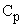is the power factor which is a nonlinear function of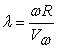where R is the radius of the blades and ω is the angular speed of the turbine blades.is usually approximated aswhere α, β, γ are constructive parameters for a given wind turbine.

According to physical and mathematical equations of the system, the generation torque of the wind turbine could be obtained as below :(2)

The dynamics of the whole system (turbine plus generator) are related to the total moment of inertia. Thus ignoring torsion in the shaft, electric dynamics of the generator and other higher order effects, the approximate system’s dynamic model is:(3)

The input to our wind energy conversion system is a time-varying wind speed. In this paper the wind speed changes assumed is shown in Figure 1:

Now the need for a controller which satisfies the following conditions arises. Reduce steady state error of the output signal Reject disturbance. The open loop system response depicted in Figure 2, shows the vital need of advanced controller for reducing error and convergence increment. One of the simplest controllers that seem to be capable of satisfying all the above conditions is a Lead-Lag controller.

### 3. HBMO Algorithm

The honey bee is a social insect that can survive only as a member of a community, or colony. The colony inhabits an enclosed cavity. A colony of honey bees consist of a queen, several hundred drones, 30,000 to 80,000 workers and broods during the active season. A colony of bees is a large family of bees living in one bee-hive. The queen is the most important member of the hive because she is the one that keeps the hive going by producing new queen and worker bees . Drones' role is to mate with the queen. Tasks of worker bees are several such as: rearing brood, tending the queen and drones, cleaning, regulating temperature, gather nectar, pollen, water, etc. Broods arise either from fertilized (represents queen or worker) or unfertilized (represents drones) eggs. The HBMO Algorithm is the combination of several different methods corresponded to a different phase of the mating process of the queen. In the marriage process, the queen(s) mate during their mating flights far from the nest. A mating flight starts with a dance performed by the queen who then starts a mating flight during which the drones follow the queen and mate with her in the air. In each mating, sperm reaches the spermatheca and accumulates there to form the genetic pool of the colony. The queen’s size of spermatheca number equals to the maximum number of mating of the queen in a single mating flight is determined. When the queen mates successfully, the genotype of the drone is stored. At the start of the flight, the queen is initialized with some energy content and returns to her nest when her energy is within some threshold from zero or when her spermatheca is full. In developing the algorithm, the functionality of workers is restricted to brood care, and therefore, each worker may be represented as a heuristic which acts to improve and/or take care of a set of broods. A drone mates with a queen probabilistically using an annealing function as :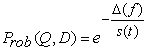(4)

Where Prob (Q, D) is the probability of adding the sperm of drone D to the spermatheca of queen Q (that is, the probability of a successful mating); ∆ (f) is the absolute difference between the fitness of D (i.e., f (D)) and the fitness of Q (i.e., f (Q)); and S (t) is the speed of the queen at time t. It is apparent that this function acts as an annealing function, where the probability of mating is high when both the queen is still in the start of her mating–flight and therefore her speed is high, or when the fitness of the drone is as good as the queen’s. After each transition in space, the queen’s speed, S(t), and energy, E(t), decay using the following equations: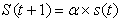(5)(6)

where α is a factor and γ is the amount of energy reduction after each transition. Also, Algorithm and computational flowchart of HBMO method to optimize the WECS controller parameters is presented in Figure 4.

Thus, HBMO algorithm may be constructed with the following five main stages:

A. The algorithm starts with the mating–flight, where a queen (best solution) selects drones probabilistically to form the spermatheca (list of drones). A drone is then selected from the list at random for the creation of broods.

B. Creation of new broods by crossoverring the drones’ genotypes with the queen’s.

C. Use of workers (heuristics) to conduct local search on broods (trial solutions).

D. Adaptation of workers’ fitness based on the amount of improvement achieved on broods.

E. Replacement of weaker queens by fitter broods.

### 4. Controller Designing Based on HBMO

Due to develop of system controllers, the conventioal contreollers are used widely in power system applications. making applicable of conventional controllers is simple againist the new controllers of power systems . The Lead-Lag controllers are widely used in most cases of power systems controllers which compensat very well. One of the most benefits of these controllers is the easily implemention in analog and digital systems. If these controllers are designed optimaly, indubitable they become one of the most implimanted controllers in modern systems. This paper introduce a new optimal Lead-Lag controller, which is used of HBMO algorithm to designing the controller of wind energy conversion system in order to control of output power. The overall controller schematic is shown in Figure 4.

Lead-Lag general controller is expressed in equation (7) which the controller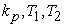parameters should be optimized using the proposed algorithm. In the load variations, it is obvious that the transient mode of the WECS system depends on the controller parameters. The conventional controller designing method are not viable to be implemented because this system is an absolute nonlinear system. So these methods would have not efficient performance in the system.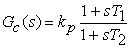(7)

In order to design controller optimal controller using HBMO for the WECS from the speed vaiation curve, we consider the worst condition for load design controllers for these conditions. Figure 5, depicts the worst condition for wind variation in the system.

At first, problem should be written as an optimization problem and then by appliyning the proposed optimization method, the best Lead-Lag controller is achieved. Selecting objective function is the most important part of this optimization problem. Because, choosing different objective functions may completely change the particles variation state. In optimization problem we considered the power error signal in order to achieved the best controller.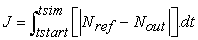(8)

Where, tsim is the simulation time in which objective function is calculated, theis the real speed of turbine andis the reference speed signal. We are reminded that whatever the objective function is a small amount in this case the answer will be more optimized. Each optimizing problem is optimized under a number of constraints. At this problem constraints should be expressed as .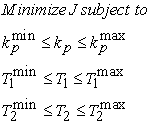(9)

Where,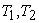are in the interval [0.01 10] and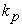in the interval [1 500].

In this problem, the number of particles, dimension of the particles, and the number of repeatations are selected 40, 3, 80, respectively. After optimization, results are determined as below: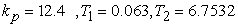(10)

### 5. Simulation Results

The conventional PID controller has initial and random values in proportional, integral and derivative coefficients as below: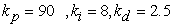(11)

Closed loop system response to the input shown in Figure 1, in the absence of disturbance is plotted in Figure 6. According to Figure 6, Proposed PID controller gives a satisfactory closed loop response when there is no disturbance. In order to check the capability of the designed Proposed PID controller in the presence of disturbance, a major disturbance on the firing angle of the thyristor at 35 seconds is applied (See Figure 7).

In order to qualify the proposed controller, closed loop system responses to the input shown in Fig 3, in the absence of disturbance and uncertainty, with disturbance applied to firing angle of the thyristor, with disturbance applied to output speed of the given turbine and with parametric uncertainty are depicted in Figs 8 through 12 respectively. It is also desired to check whether the proposed controller is capable of rejecting disturbances and being robust to uncertainties simultaneously. Fig.13 depict corresponding control signal in the presence of both the disturbance and the uncertainty. According to Figs 8 through 13 one can say that the proposed controller is much more effective than conventional PID controller. The proposed controller offers a fast response with almost zero overshoot and is very operational in rejecting disturbances. It could be easily seen that our controller behaves effectively and pretty fast when a disturbance is applied. It also operates pretty well when there are parametric uncertainties.

Figure 12. Control signal with both the disturbance and the uncertainty

### 6. Conclusion

In this paper, a novel method to control the WECS was proposed. It was shown that these proposed methods, can explain instinct nonlinear behavior turbine/ generator systems very well. At first, the conventional PID controller is used in order to control of WECS speed. It was seen that the proposed controller offered a desired closed loop response when the system was subjected to major external disturbance. Then, proposed method, which was used the HBMO optimization algorithm controller, was applied to control the WECS. Issues such as external disturbances which are associated with all practical systems ask for model-free. The proposed controller not only increases the closed system band width but also offers a desired response when the system is subjected to various disturbances. The high adaptation speed along with high stability and performance characteristics make this method a high quality control approach.

### References

  K.Ogawa, N.Ymammura, M.Ishda, “Study for Small Size Wind Power Generating System Using Switched Reluctance Generator”, IEEE International Conference on Industrial Technology, pp. 1510-1515, 2006.In article  F. D. Bianchi, H. De Battista and R. J. Mantz, “Wind Turbine Control Systems Principles, Modeling and Gain Scheduling Design” Springer-Verlag London Limited 2007.In article  Miguel Angel Mayosky, Gustavo I.E.Cancelo “Direct Adaptive Control of Wind Energy Conversion Systems Using Gaussian Networks” IEEE Trans on Neural Networks, Vol. 10, pp.898-906, July 1999.In article CrossRef  M.Sedighizade, A.Rezazadeh “Adaptive PID Control of Wind Energy Conversion Systems Using RASP1 Mother Wavelet Basis Function Networks” Proceedings of Academy of Science, Engineering and Technology Vol.27, pp. 269-273. February 2008.In article  M.Sedighizade “Nonlinear Model Identification and Control of Wind Turbine Using Wavelets" Proceedings of the 2005 IEEE Conference on Control Applications Toronto, pp. 1057-1062 Canada, 2005.In article  M. Kalantari, M. Sedighizadeh “Adaptive Self Tuning Control of Wind Energy Conversion Systems Using Morlet Mother Wavelet Basis Functions Networks”12th Mediterranean IEEE Conference on Control and Automation MED’04, Kusadasi, Turkey, 2004.In article  X. Zhang, D. XU and Y. LIU, “Predictive Functional Control of a Doubly Fed Induction Generator for Variable Speed Wind Turbines,” 5th World Congress on Intelligent Control and Automation, June 15-19, Hangzhou. P.R. China, 2004.In article  G. A. Smith, Power electronics for recovery of wind and solar energy, Wind Eng., Vol. 19, no. 2, 1995.In article  M. Boutoubat, L. Mokrani, M. Machmoum “Control of a wind energy conversion system equipped by a DFIG for active power generation and power quality improvement” Renewable Energy 50 (2013) 378e386.In article  Noradin Ghadimi “Genetically Adjusting of PID Controllers Coefficients for Wind Energy Conversion System with doubly fed induction Generator” World Applied Sciences Journal 15 (5), 702-707, 2011.In article  Shuai Xiao, Hua Geng, Honglin Zhou, Geng Yang” Analysis of the control limit for rotor-side converter of doubly fed induction generator-based wind energy conversion system under various voltage dips” IET Renew. Power Gener., 2013, Vol. 7, Iss. 1, pp. 71-81.In article CrossRef  P.Puleston, “Control strategies for wind energy conversion systems”, Ph.D. dissertation, Univ. La Plata, Argentina, 1997.In article  Yannis M, Magdalene M, Georgios D (2011). “Honey bees mating optimization algorithm for the Euclidean traveling salesman problem,” Infor. Sci. 181(20):4684-4698.In article CrossRef  Niknam T (2011). An efficient multi-objective HBMO algorithm for distribution feeder reconfiguration. Expert Syst. Appl. 38(3): 2878-2887.In article CrossRef